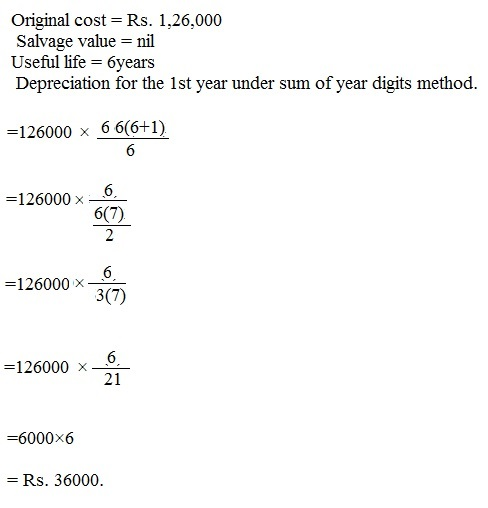# What is Sum Of The Years ?

### What are ‘Sum-Of-The-Years’ Digits ‘

An accelerated method for calculating an asset’s depreciation. This method takes the asset’s expected life and adds together the digits for each year. So if the asset was expected to last for five years, the sum of the years’ digits would be obtained by adding: 5 + 4 + 3 + 2 + 1 to get a total of 15. Each digit is then divided by this sum to determine the percentage by which the asset should be depreciated each year, starting with the highest number in year 1.

### BREAKING DOWN ‘Sum-Of-The-Years’ Digits ‘

It makes sense to use an accelerated depreciation method such as the SYR method when an asset will lose most of its value toward the beginning of its useful life – as is the case with automobiles, for example.
In the five year example above, the SYD method would yield the following depreciation schedule:

Year 1: 5/15 = 33%

Year 2: 4/15 = 27%

Year 3: 3/15 = 20%

Year 4: 2/15 = 13%

Year 5: 1/15 = 7%

The percentages for each year should add up to 100%.

#### Question:

Original cost =Rs.1,26,000: Salvage value = nil: Useful life = 6 years.Depreciation for the 1st year under sum of years digits method will be

a. Rs.6,000

b. Rs.12,000

c. Rs.18,000

d. Rs.36,000

#### Explanation: